## Example Questions

← Previous 1 3 4 5

### Example Question #1 : How To Find The Surface Area Of A Cylinder

The area of the base of a circular right cylinder is quadrupled. By what percentage is the outer face increased by this change?

250%

400%

200%

300%

100%

100%

Explanation:

The base of the original cylinder would have been πr2, and the outer face would have been 2πrh, where h is the height of the cylinder.

Let's represent the original area with A, the original radius with r, and the new radius with R: therefore, we know πR2 = 4A, or πR24πr2. Solving for R, we get R = 2r; therefore, the new outer face of the cylinder will have an area of 2πRh or 2π2rh or 4πrh, which is double the original face area; thus the percentage of increase is 100%. (Don't be tricked into thinking it is 200%. That is not the percentage of increase.)

### Example Question #2 : Cylinders

What is the surface area of a cylinder with a radius of 17 and a height of 3?

2137

2205

3107

1984

2000

2137

Explanation:

We need the formula for the surface area of a cylinder: SA = 2πr2 + 2πrh. This formula has π in it, but the answer choices don't. This means we must approximate π. None of the answers are too close to each other so we could really even use 3 here, but it is safest to use 3.14 as an approximate value of π.

Then SA = 2 * 3.14 * 172 + 2 * 3.14 * 17 * 3 ≈ 2137

### Example Question #3 : Cylinders

What is the surface area of a cylinder with a radius of 6 and a height of 9?

64π

225π

180π

96π

108π

180π

Explanation:

surface area of a cylinder

= 2πr2 + 2πrh

= 2π * 62 + 2π * 6 *9

= 180π

### Example Question #1 : How To Find The Surface Area Of A Cylinder

Quantitative Comparison

Quantity A: The volume of a cylinder with a radius of 3 and a height of 4

Quantity B: 3 times the volume of a cone with a radius of 3 and a height of 4

Quantity A is greater.

The two quantities are equal.

The relationship cannot be determined from the information given.

Quantity B is greater.

The two quantities are equal.

Explanation:

There is no need to do the actual computations here to find the two volumes. The volume of a cone is exactly 1/3 the volume of a cylinder with the same height and radius. That means the two quantities are equal. The formulas show this relationship as well: volume of a cone = πr2h/3 and volume of a cylinder = πr2h

### Example Question #1501 : Gre Quantitative Reasoning

A right circular cylinder of volume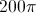has a height of 8.

Quantity A: 10

Quantity B: The circumference of the base

Quantity A is greater

The two quantities are equal

Quantity B is greater

The relationship cannot be determined from the information provided.

Quantity B is greater

Explanation:

The volume of any solid figure is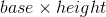. In this case, the volume of the cylinder isand its height is, which means that the area of its base must be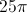. Working backwards, you can figure out that the radius of a circle of areais. The circumference of a circle with a radius ofis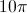, which is greater than.

### Example Question #6 : Cylinders

What is the surface area of a cylinder that has a diameter of 6 inches and is 4 inches tall?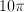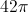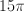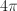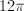Explanation:

The formula for the surface area of a cylinder is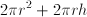,

whereis the radius and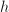is the height.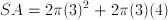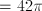### Example Question #1 : Solid Geometry

A cylinder has a radius of 4 and a height of 8.  What is its surface area?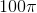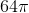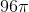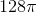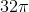Explanation:

This problem is simple if we remember the surface area formula!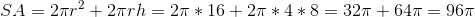### Example Question #8 : Cylinders

Quantitative Comparison

Quantity A: Surface area of a cylinder that is 2 feet high and has a radius of 4 feet

Quantity B: Surface area of a box that is 3 feet wide, 2 feet high, and 4 feet long

The two quantities are equal.

The relationship cannot be determined from the information given.

Quantity B is greater.

Quantity A is greater.

Quantity A is greater.

Explanation:

Quantity A: SA of a cylinder = 2πr2 + 2πrh = 2π * 16 + 2π * 4 * 2 = 48π

Quantity B: SA of a rectangular solid = 2ab + 2bc + 2ac = 2 * 3 * 2 + 2 * 2 * 4 + 2 * 3 * 4 = 52

48π is much larger than 52, because π is approximately 3.14.

### Example Question #1 : Solid Geometry

A cylinder has a height of 4 and a circumference of 16π. What is its volume

64π

16π

256π

128π

none of these

256π

Explanation:

circumference = πd

d = 2r

volume of cylinder = πr2h

r = 8, h = 4

volume = 256π

### Example Question #1 : How To Find The Volume Of A Cylinder

Rusty is considering making a cylindrical grain silo to store his crops. He has an area that is 6 feet long, 6 feet wide and 12 feet tall to build a cylinder in. What is the maximum volume of grain that he can store in this cylinder?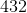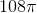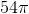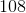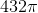Explanation:

The maximum cylindrical base can have a diameter of 6 and therefore a radius of 3. The formula for the volume of a cylinder is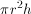, which in this case is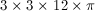← Previous 1 3 4 5

Tired of practice problems?

Try live online GRE prep today.